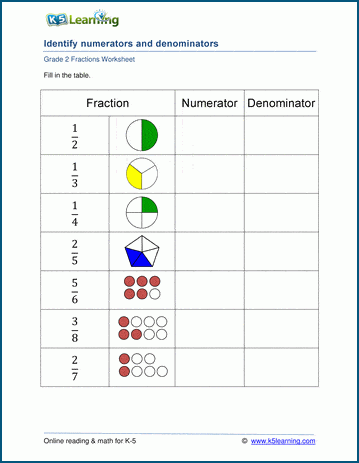# Numerators and denominators

## Fraction worksheets: identifying numerators and denominators

These grade 2 math worksheets introduce students to numerators ("top number" in fraction), denominators ("bottom number") and the standard form of writing fractions.  Students are given a basic fraction in standard form, with an image, and asked to write down the numerator and denominator.## More fractions worksheets

Explore all of our fractions worksheets, from dividing shapes into "equal parts" to multiplying and dividing improper fractions and mixed numbers.

## What is K5?

K5 Learning offers reading and math worksheets, workbooks and an online reading and math program for kids in kindergarten to grade 5.  We help your children build good study habits and excel in school.